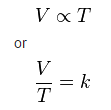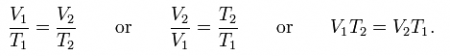Chemistry

# What is Charles’s Law?

Charles’s Law:
At constant pressure, the volume of a confined gas is directly proportional to the absolute temperature.

V = kT

Charles’s law is an experimental gas law which describes how gases tend to expand when heated. A modern statement of Charles’s law is “When the pressure on a sample of a dry gas is held constant, the Kelvin temperature and the volume will be directly related.

This directly proportional relationship can be written as:where:

V is the volume of the gas
T is the temperature of the gas (measured in Kelvin).
k is a constant.

This law describes how a gas expands as the temperature increases; conversely, a decrease in temperature will lead to a decrease in volume. For comparing the same substance under two different sets of conditions, the law can be written as:The equation shows that, as absolute temperature increases, the volume of the gas also increases in proportion.

Charles’s Law Formula :

• Gas Equation: Vi/Ti = VfTf
• Initial Volume(Vi) = VfTi / Tf
• Initial Temperature(Ti) = ViTf / Vf
• Final Volume(Vf) = ViTf / Ti
• Final Temperature(Tf) = VfTi / Vi

where, Vi = Initial Volume, Ti = Initial Temperature, Vf = Final Volume. Tf = Final Temperature.